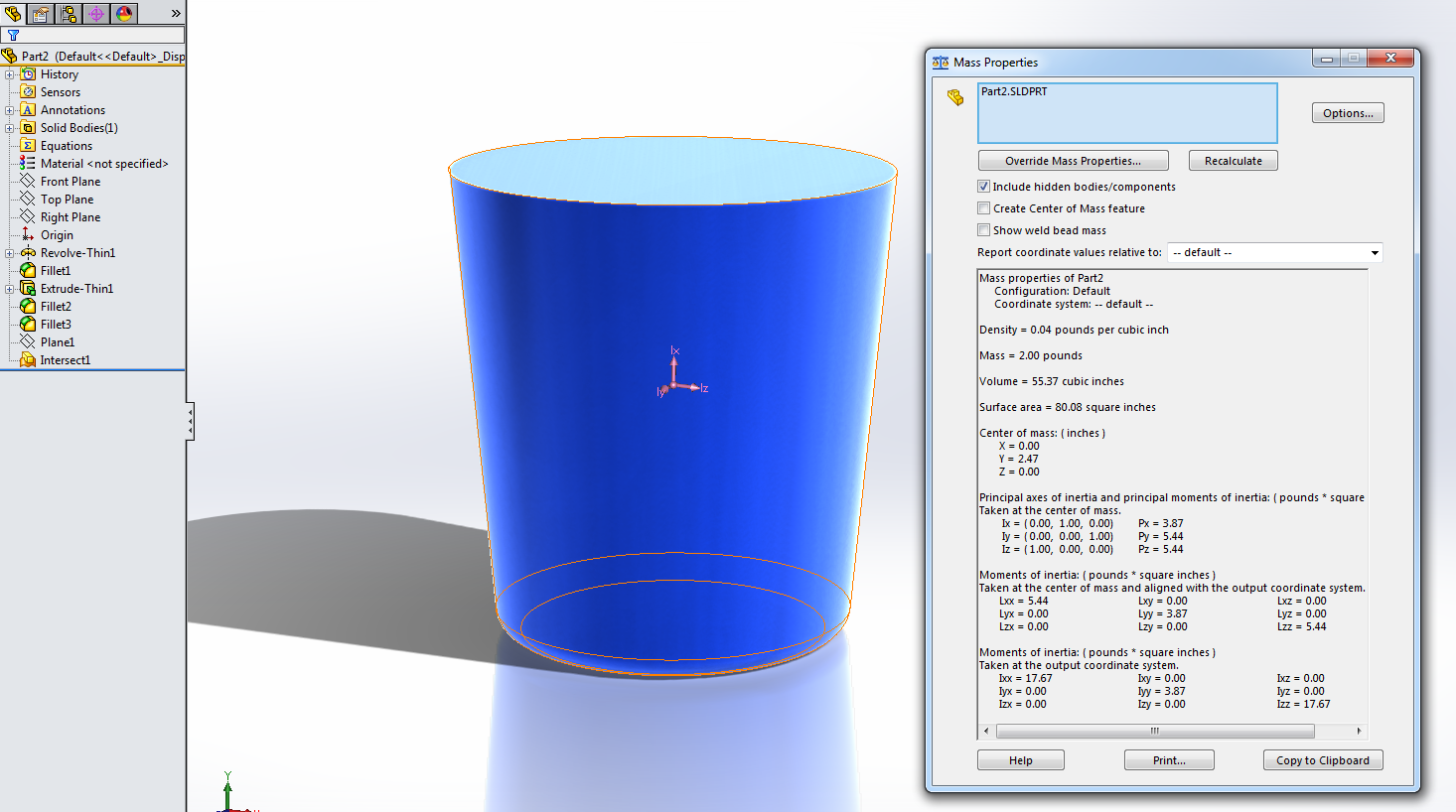# How can we calculate the volume of a drawn model using SolidWorks ?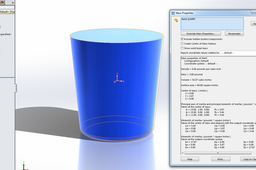Richard and Kaiwei: This process is actually pretty easy in SOLIDWORKS (as of version 2014). See the list below:

1. ### Step 1:

I created a basic coffee mug as an example here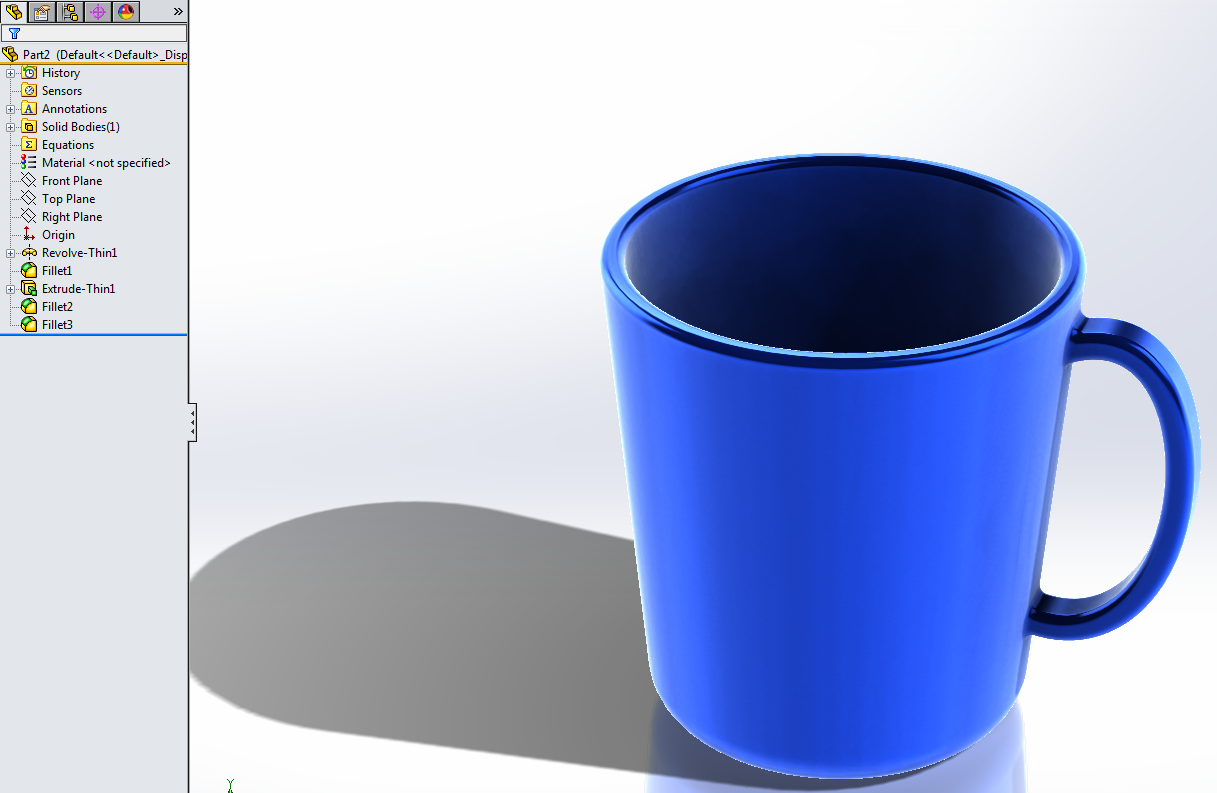2. ### Step 2:

Create a reference plane that cuts through the part. In this case, I estimated where the coffee cup would be "filled up" to.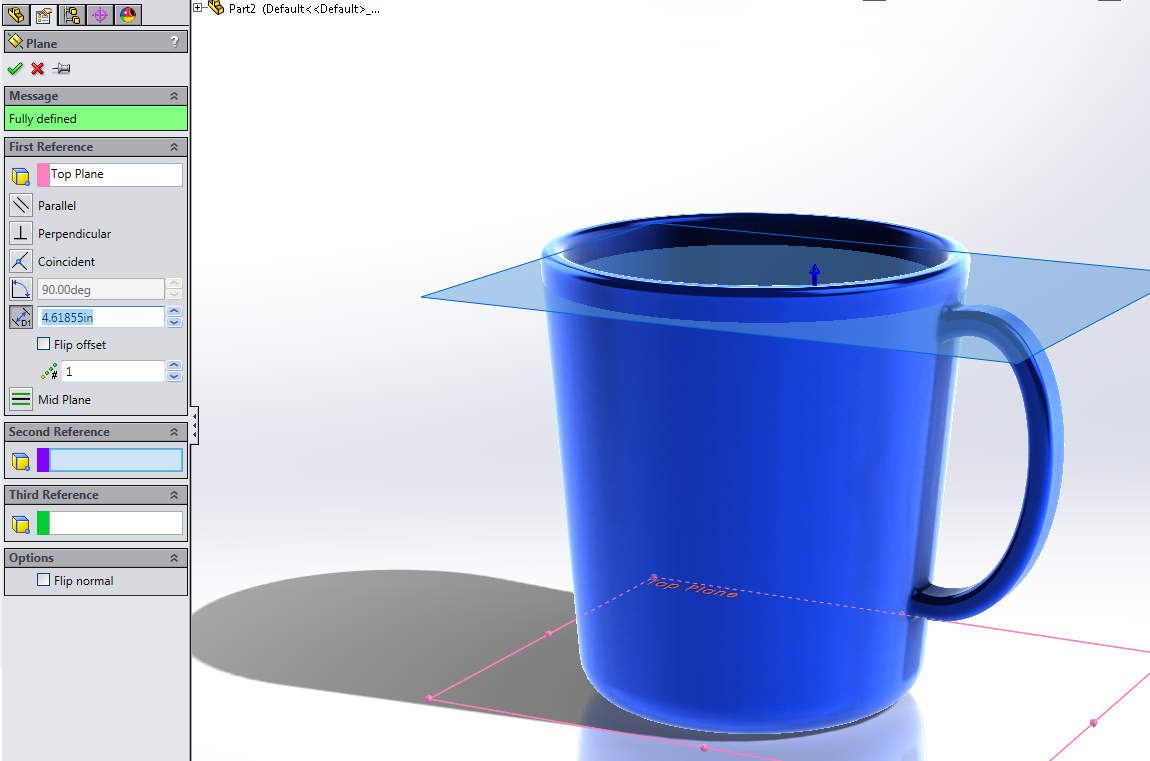3. ### Step 3:

After the plane is created, fire up the "Intersect" feature, and select the reference plane and the solid body.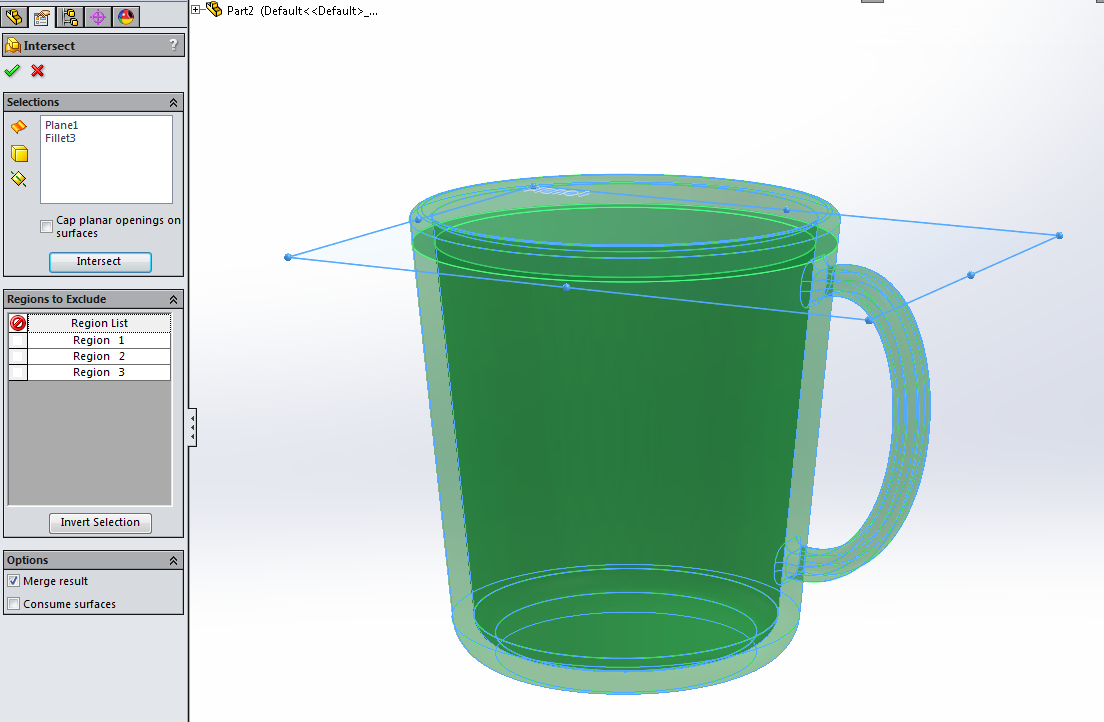4. ### Step 4:

Exclude the regions not needed, in most cases - those regions will be 1 and 3. The three listed regions here are all the possible bodies that can be created using the solid body and the reference plane I created.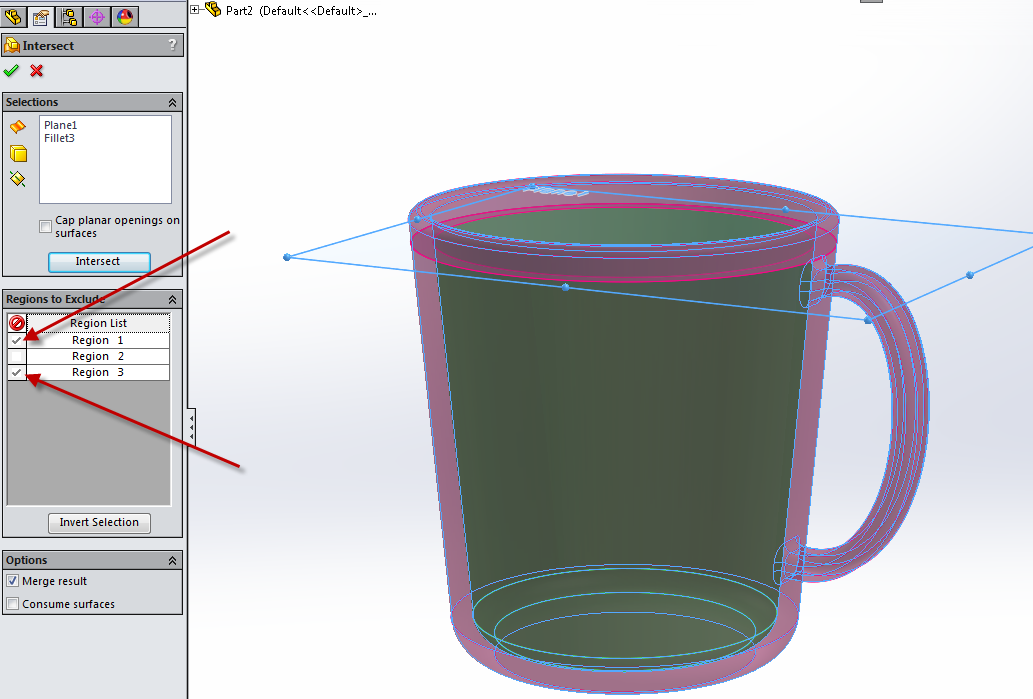5. ### Step 5:

Final step is to reference the above steps (from MI Ilmy) to calculate the volume of what's left (the internal volume of the coffee cup)